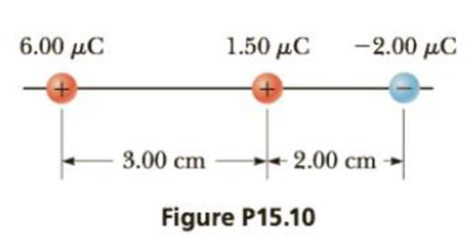Chapter 15, Problem 10P

Chapter
Section
Textbook Problem

Calculate the magnitude and direction of the Coulomb force on each of the three charges shown in Figure P15.10.To determine
The magnitude and direction of Coulomb force on each of the three charges.

Explanation

The force diagram is given by,

In the above diagram,

• Fq1 is the force due to q1 .
• Fq2 is the force due to q2 .
• Fq3 is the force due to q3 .

From Coulomb’s law, the force between two charges is given by,

F=keQ1Q2r2

• ke is the Coulomb constant.
• Q1 and Q2 are the charges.
• r is the distance of separation.

Formula to calculate the force on q1 is,

F1=Fq2Fq3=(keq1q2r122)(keq1q3r132)

• r12 is the distance between q1 and q2 .
• r13 is the distance between q1 and q3 .

Substitute 8.99×109N.m2/C2 for ke , 6μC for q1 , 1.5μC for q2 , 2μC for q3 , 3.00 cm for r12 and 5.00 cm for r13 .

F1=(8.99×109N.m2/C2)(6μC)(1.5μC(3.00cm)2)(2μC(5.00cm)2)=(8.99×109N.m2/C2)(6×106C)(1.5×106C(3.00×102m)2)(2×106C(5.00×102m)2)=46.7N

Formula to calculate the force on q2 is,

F2=Fq1+Fq3=(keq1q2r122)+(keq2q3r232)

• r12 is the distance between q1 and q2 .
• r23 is the distance between q2 and q3 .

Substitute 8.99×109N.m2/C2 for ke , 6μC for q1 , 1

Still sussing out bartleby?

Check out a sample textbook solution.

See a sample solution

The Solution to Your Study Problems

Bartleby provides explanations to thousands of textbook problems written by our experts, many with advanced degrees!

Get Started

A represents a quantitative observation.

Introductory Chemistry: A Foundation

What is the structure and function of the extracellular matrix?

Biology: The Dynamic Science (MindTap Course List)

Which class has the largest individuals? Which is the most economically important?

Oceanography: An Invitation To Marine Science, Loose-leaf Versin